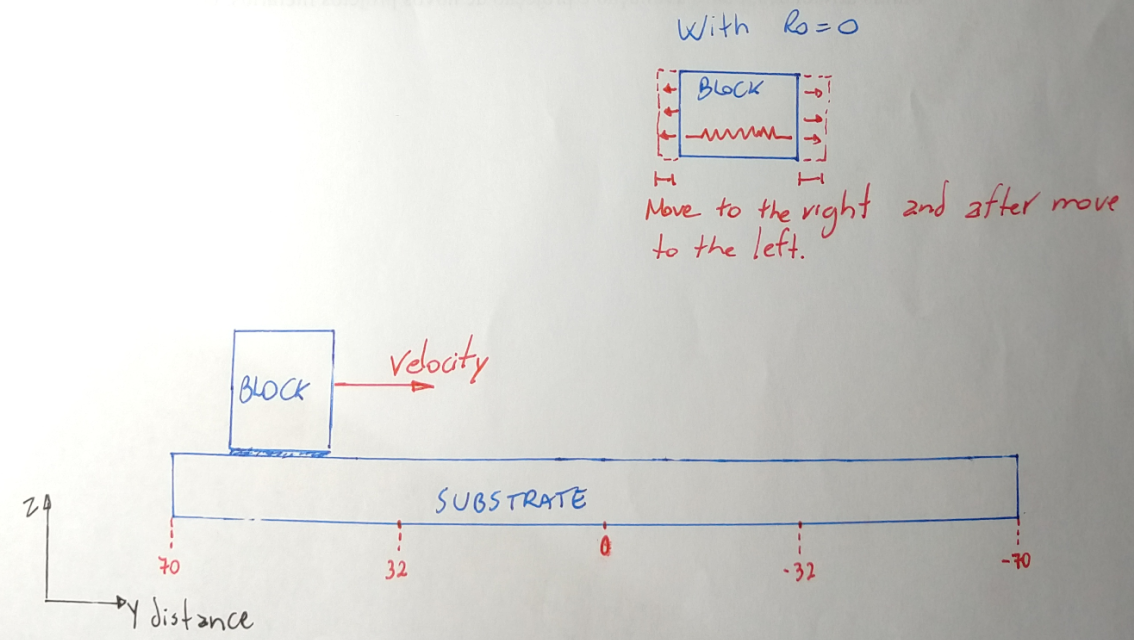# Fix smd -- understanding the syntax

Hi

I am trying to simulate a superior block (similar to a tip), sliding on a surface with constant velocity.

For this, I am using the fix smd command to put a spring between the center of mass of the superior block and a position y. The idea is to pull the block toward the position y, extracting the y-component of the pulling force (position 2 of the vector that storage the quantities).

Brief visualization the system (attached is a schematic figure to clarify):
[BLOCK]<–////–><--------------------->[FIXED POINT]1 Like

The mechanics of fix smd is best understood with some simple examples. Please see below a simple system with just one particle with no interactions except fix smd at rest placed at -5.0\AA 0.0\AA 0.0\AA and a fix smd in tether mode attached to 5.0\AA 0.0\AA 0.0\AA with cvel setting of -0.1\AA/fs.
That means, the particle and the reference point are initially 10\AA apart and that distance is decremented by 0.01 (due to using a 0.1 fs time step) in every step.
You can see from the thermo log, how the particle is picking up velocity and will follow the steering force from fix smd and after some steps will oscillate around the moving reference point. the best was to visualize this is that you have the pulled particle attached to a stick of continuously decreasing length with a spring that is of length R0 at rest (i.e. 0.0 in this example). for a larger group of particles, the reference is the center of mass of that group. so if you replace the one atom with two atoms of half the mass at equal distance from 5.0 0.0 0.0 and attach fix smd to the those two in the same way, you will get the same center of mass motion as a result. please see the correspondingly modified version of the single particle input example below.

HTH,
Axel.

1 particle example:

units real
atom_modify map array
region box block -10 10 -10 10 -10 10
create_box 1 box

create_atoms 1 single -5.0 0.0 0.0
mass 1 1.0
pair_style zero 1.0
pair_coeff 1 1
timestep 0.1

group pull type 1

fix pull pull smd cvel 100.0 -0.1 tether 5.0 0.0 0.0 0.0
fix move all nve
variable xpos equal x
variable xfor equal fx
variable xvel equal vx
variable sfor equal f_pull
variable slen equal f_pull
variable sdist equal f_pull

thermo_style custom step v_xpos v_xvel v_xfor v_sfor v_slen v_sdist
thermo 1

run 1000

Two particle example:

units real
atom_modify map array
region box block -10 10 -10 10 -10 10
create_box 1 box

create_atoms 1 single -5.5 0.0 0.0
create_atoms 1 single -4.5 0.0 0.0
mass 1 0.5
pair_style zero 1.0
pair_coeff 1 1
timestep 0.1

group pull type 1

fix pull pull smd cvel 100.0 -0.1 tether 5.0 0.0 0.0 0.0
fix move all nve
variable xpos equal (x+x)*0.5
variable xfor equal fx+fx
variable xvel equal (vx+vx)*0.5
variable sfor equal f_pull
variable slen equal f_pull
variable sdist equal f_pull

thermo_style custom step v_xpos v_xvel v_xfor v_sfor v_slen v_sdist
thermo 1

run 1000